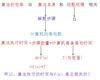S(n)=O(f(n))。

space complexity

• 1，什么是时间复杂度？ 一个问题的规模是n，解决这一问题所需算法所需要...空间复杂度需要考虑在运行过程中为局部变量分配的存储空间的大小，它包括为参数表中形参变量分配的存储空间和为在函数体中定义的局部变量分配
• 算法复杂度分为时间复杂度和空间复杂度。 其作用： 时间复杂度是指执行算法所需要的计算工作量； 而空间复杂度是指执行这个算法所需要的内存空间。 （算法的复杂性体现在运行该算法时的计算机所需资源的多少上，...
• 不要使用额外的数组空间，你必须在 原地 修改输入数组 并在使用 O(1) 额外空间的条件下完成。 if(nums.length < 1) return 0; int i = 0; for(int j=1; j<nums.length; j++) { if(!(nums[i] == nums...
leetcode 26 – 删除排序数组中的重复项 给定一个排序数组，你需要在 原地 删除重复出现的元素，使得每个元素只出现一次，返回移除后数组的新长度。
不要使用额外的数组空间，你必须在 原地 修改输入数组 并在使用 O(1) 额外空间的条件下完成。
if(nums.length < 1) return 0;
int i = 0;
for(int j=1; j<nums.length; j++) {
if(!(nums[i] == nums[j])) {
nums[i+1] = nums[j];
i++;
}
}
return i+1;

复杂度也叫渐进复杂度，包括时间复杂度和空间复杂度，用来分析算法执行效率与数据规模之间的增长关系，可以粗略地表示，越高阶复杂度的算法，执行效率越低。
空间复杂度： 全称是 渐进空间复杂度，表示算法的存储空间与数据规模之间的增长关系。 时间复杂度： 全称是 渐进时间复杂度，表示算法的执行时间与数据规模之间的增长关系。
1、空间复杂度
一个程序的空间复杂度是指运行完一个程序所需内存的大小。 利用程序的空间复杂度，可以对程序的运行所需的内存有个预先估计。
程序执行时所需存储空间包括以下两部分：
固定部分。这部分空间的大小与输入/输出的数据的个数多少、数值无关。主要包括指令空间（即代码空间）、数据空间（常量、简单变量）等所占的空间。这部分属于静态空间。  可变空间。这部分空间主要包括动态分配的空间，以及递归栈所需的空间等。这部分的空间大小与算法有关。
我们在写代码时，完全可以用空间来换取时间，比如字典树、哈希等都是这个原理。HashMap.get()、put()都是O(1)的时间复杂度。
空间复杂度为O(1)：有的算法只需要占用少量的临时工作单元，而且不随问题规模的大小而改变，我们称这种算法是“就地”执行的，是节省存储的算法，空间复杂度为O(1)。
空间复杂度为O(n)：有的算法需要占用的临时工作单元与解决问题的规模n有关，它随着n的增大而增大，当n较大时，将占用较多的存储单元，例如快速排序和归并排序算法就属于这种情况。
常见的空间复杂度就是O(1)、O(n)、O(n^2)，像是O(logn)、O(nlogn)对数阶的复杂度平时都用不到，而且空间复杂度分析比时间复杂度分析要简单很多。
（1）空间复杂度为：O(1)
int i = 1;
int j = 2;
++i;
j++;
int m = i + j;

代码中的i、j、m所分配的空间都不随着处理数据量变化，因此它的空间复杂度S(n) = O(1)
（2）空间复杂度：O(n)
void print(int n) {
int i = 0;
int[] a = new int[n];
for (i; i <n; ++i) {
a[i] = i * i;
}
}

第三行new了一个数组，这个数组占用的大小为n，虽然后面有循环，但是没有再分配新的空间，因此空间复杂度S(n) = O(n)。
2、时间复杂度
（1）T(n) = O(2n+2) (看不懂没关系，后面有解释) 时间复杂度：O(n)
int cal(int n) {
int sum = 0;
int i = 1;
for (; i <= n; ++i) {
sum = sum + i;
}
return sum;
}


假设每行代码的执行时间都是一样的，为unit_time单位时间。
第 2、3 行代码分别需要== 1 个 unit_time== 的执行时间，第 4、5 行都运行了 n 遍，所以需要 2n*unit_time 的执行时间，所以这段代码总的执行时间就是== (2n+2)*unit_time==。可以看出来，所有代码的执行时间 T(n) 与每行代码的执行次数成正比。
（2）T(n) = O(2n2+ 2n + 3) 时间复杂度：O(n^2)
int cal(int n) {
int sum = 0;
int i = 1;
int j = 1;
for (; i <= n; ++i) {
j = 1;
for (; j <= n; ++j) {
sum = sum +  i * j;
}
}
}


第 2、3、4 行代码，每行都需要 1 个 unit_time 的执行时间，第 5、6 行代码循环执行了 n 遍，需要 2n * unit_time 的执行时间，第 7、8 行代码循环执行了 n2遍，所以需要 2n2 * unit_time 的执行时间。所以，整段代码总的执行时间 T(n) = (2n2+ 2n + 3)*unit_time。
规律：所有代码的执行时间T(n)与每行代码的执行次数成正比 把规律总结成一个公式： T(n) = O(f(n)) 其中，f(n)表示每行代码执行的次数总和，因为是一个公式，所以用f(n)来表示； O表示代码的执行时间T(n)与f(n)表达式成正比。
上面是大O时间复杂度的由来和表示方法，但是分析代码的时间复杂度，一般有三种方式：（1）只关注循环执行次数最多的一段代码；（2）总的时间复杂度就等于量级最大的那段代码的时间复杂度；（3）嵌套代码的复杂度等于嵌套内外代码复杂度的乘积。
几种常见的时间复杂度实例 1、常量阶 O(1)O(1)O(1) 2、对数阶 O(logn)O(logn)O(logn) 3、线性阶 O(n)O(n)O(n) 4、线性对数阶 O(nlogn)O(nlogn)O(nlogn) 5、平方阶 O(n2)、立方阶 O(n3)……k 次方阶 O(nk) 6、指数阶 O(2n) 7、阶乘阶 O(n!)
（1）O(1)
int a = 1;
int b = 2;
int c = 3;

一般情况下，只要算法中不存在循环语句、递归语句，即使有成千上万行的代码，其时间复杂度也是Ο(1)。 （2）O(logn)、O(nlogn)
 i=1;
while (i <= n)  {
i = i * 2;
}

执行次数为log2n，所以，这段代码的时间复杂度为O(log2n)
 i=1;
while (i <= n)  {
i = i * 3;
}

执行次数为log3n，所以，这段代码的时间复杂度为O(log3n) 实际上，不管是以2为底、以3为底还是以10为底，我们可以把所有对数阶的时间复杂度都记为O(logn)，忽略对数的底。
for(m = 1; m < n; m++) {
i = 1;
while(i < n) {
i = i * 2;
}
}

上述的时间复杂度为O(nlogn)
（3）O(m+n)、O(m*n) 代码的复杂度由两个数据的规模来决定
int cal(int m, int n) {
int sum_1 = 0;
int i = 1;
for (; i < m; ++i) {
sum_1 = sum_1 + i;
}

int sum_2 = 0;
int j = 1;
for (; j < n; ++j) {
sum_2 = sum_2 + j;
}

return sum_1 + sum_2;
}

代码中，m和n表示两个数据规模，我们无法事先评估m和n谁的量级大，所以上面代码的时间复杂度就是O(m+n)。
（4）O(n)
for(i=1; i<=n; i++) {
j = i;
j++;
}

（5）O(n^2)
for(x=1; i <= n; x++){
for(i = 1; i <= n; i++) {
j = i;
j++;
}
}

二分查找的时间复杂度：logn https://www.cnblogs.com/yellowgg/p/11272908.html
参考： https://juejin.cn/post/6844904167824162823 https://juejin.cn/post/6844904087876534280
展开全文数据结构 java
• 对java的8种排序方法的空间复杂度和时间复杂度，进行了一个简单的统计java
• 用C语言O（1）空间复杂度实现单链表反转，C语言数据结构的作业，有需要的尽管拿去用吧，赚点小分，无聊腻了C链表反转
• 主要给大家介绍了关于如何通过js示例讲解时间复杂度与空间复杂度的相关资料，文中通过示例代码介绍的非常详细，对大家学习或者使用js具有一定的参考学习价值，需要的朋友们下面来一起学习学习吧
• 时间复杂度和空间复杂度的概念及各种算法的时间复杂度 及举例 算法的复杂度可分为俩种 一种时间复杂度 另一种是空间复杂度。 俩者的概念：时间复杂度是指执行这个算法所需要的计算工作量；而空间复杂度是指执行这个...
时间复杂度和空间复杂度的概念及各种算法的时间复杂度 及举例
算法的复杂度可分为俩种 一种时间复杂度 另一种是空间复杂度。
俩者的概念：时间复杂度是指执行这个算法所需要的计算工作量；而空间复杂度是指执行这个算法所需要的内存空间。时间和空间（即寄存器）都是计算机资源的重要体现，而算法的复杂性就是体现在运行该算法时的计算机所需的资源多少。
各种算法的复杂度如下：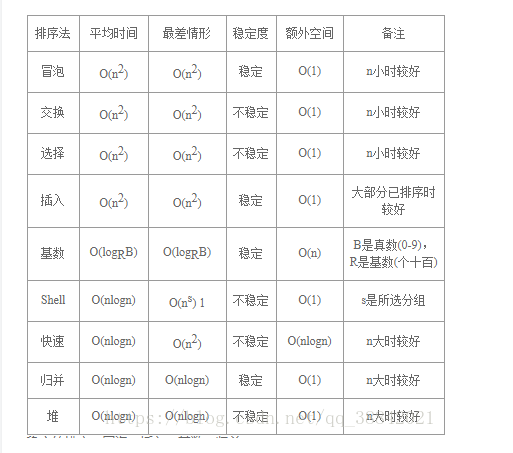时间复杂度：
1：算法的时间复杂度反映了程序执行时间随输入规模增长而增长的量级，在很大程度上能很好地反映出算法的优劣与否；
2：算法执行时间需要依据该算法编制的程序在计算机上执行运行时所消耗的时间来度量，度量方法有两种，事后统计方法和事前分析估算方法，因为事后统计方法更多的依赖计算机的硬件，软件等环境因素，有时容易掩盖算法本身的优劣。因此常常采用事前分析估算的方法；
3：一个算法是由控制结构（顺序，分支，循环三种）和原操作（固有数据类型的操作）构成的，而算法时间取决于两者的综合效率；
4：一个算法花费的时间与算法中语句的执行次数成正比，执行次数越多，花费的时间就越多。一个算法中的执行次数称为语句频度或时间频度。记为T（n）；
5：在时间频度中，n称为问题的规模，当n不断变化时，它所呈现出来的规律，我们称之为时间复杂度（其实在这中间引入了一个辅助函数f（n），但它与t（n）是同数量级函数，我们且先这样理解。）
6：在各种算法中，若算法中的语句执行次数为一个常数，则时间复杂度为o（1）；同时，若不同算法的时间频度不一样，但他们的时间复杂度却可能是一样的，eg：T(n)=n^2+2n+4 与 T（n）=4n2+n+8，他们的时间频度显然不一样，但他们的时间复杂度却是一样的，均为O(n2)，时间复杂度只关注最高数量级，且与之系数也没有关系。
7： 求解算法的时间复杂度的具体步骤是： 　　⑴ 找出算法中的基本语句； 　　算法中执行次数最多的那条语句就是基本语句，通常是最内层循环的循环体。 　　⑵ 计算基本语句的执行次数的数量级； 　　只需计算基本语句执行次数的数量级，这就意味着只要保证基本语句执行次数的函数中的最高次幂正确即可，可以忽略所有低次幂和最高次幂的系数。这样能够简化算法分析，并且使注意力集中在最重要的一点上：增长率。 　　⑶ 用大Ο记号表示算法的时间性能。 　　将基本语句执行次数的数量级放入大Ο记号中。 　　如果算法中包含嵌套的循环，则基本语句通常是最内层的循环体，如果算法中包含并列的循环，则将并列循环的时间复杂度相加 下面我来举一个简单例子：
for(i=1;i<=n;i++) {a++}; for(i=1;i<=n;i++) { for(j=1;j<=n;j++) { a++; } } 第一个for循环的时间复杂度为o（n），第二个for循环时间复杂度为o（n2），则整个算法的时间复杂度为o(n2+n)。 o(1)表示基本语句的执行次数是一个常数，一般来说，只要算法中不存在循环语句，时间复杂度就为o(1)。
空间复杂度（Space Complexity）：
1：空间复杂度是对一个算法在运行过程中临时占用存储空间大小的量度；
2：一个算法在计算机上占用的内存包括：程序代码所占用的空间，输入输出数据所占用的空间，辅助变量所占用的空间这三个方面，程序代码所占用的空间取决于算法本身的长短，输入输出数据所占用的空间取决于要解决的问题，是通过参数表调用函数传递而来，只有辅助变量是算法运行过程中临时占用的存储空间，与空间复杂度相关；
3：通常来说，只要算法不涉及到动态分配的空间，以及递归、栈所需的空间，空间复杂度通常为0(1)；
4： 对于一个算法，其时间复杂度和空间复杂度往往是相互影响的。当追求一个较好的时间复杂度时，可能会使空间复杂度的性能变差，即可能导致占用较多的存储空间；反之，求一个较好的空间复杂度时，可能会使时间复杂度的性能变差，即可能导致占用较长的运行时间。另外，算法的所有性能之间都存在着或多或少的相互影响。因此，当设计一个算法(特别是大型算法)时，要综合考虑算法的各项性能，算法的使用频率，算法处理的数据量的大小，算法描述语言的特性，算法运行的机器系统环境等各方面因素，才能够设计出比较好的算法。
展开全文• 一、空间复杂度定义 空间复杂度(Space Complexity)是对一个算法在运行过程中临时占用存储空间大小的量度。 一个算法在计算机存储器上所占用的存储空间，包括程序代码所占用的空间，输入数据所占用的空间和辅助变量所...
一、空间复杂度定义
空间复杂度(Space Complexity)是对一个算法在运行过程中临时占用存储空间大小的量度。 一个算法在计算机存储器上所占用的存储空间，包括程序代码所占用的空间，输入数据所占用的空间和辅助变量所占用的空间这三个方面。
二、影响空间复杂度的因素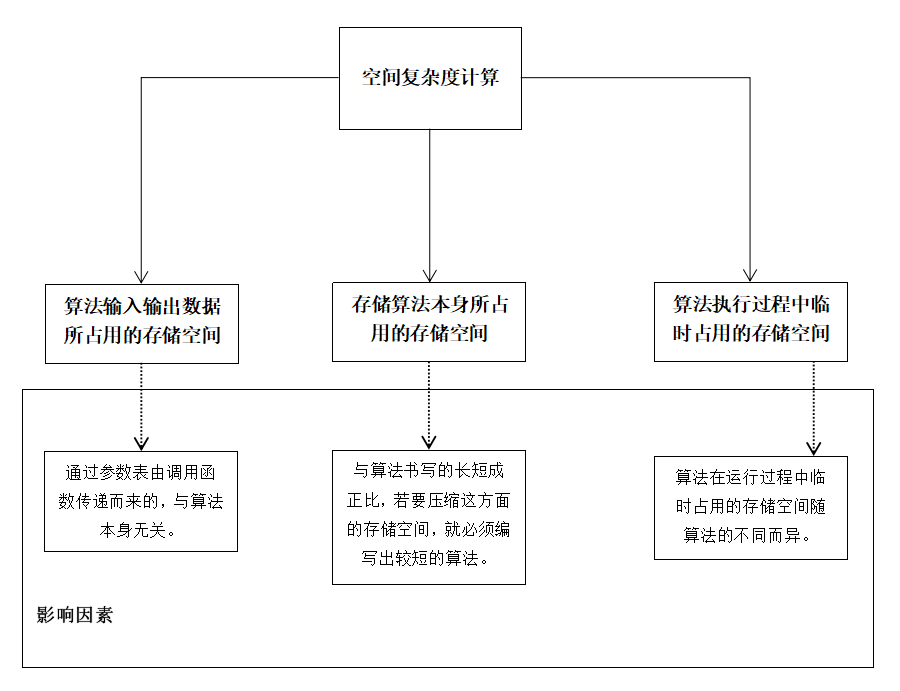注意： 一个算法的空间复杂度只考虑在运行过程中为局部变量分配的存储空间的大小，它包括为参数表中形参变 量分配的存储空间和为在函数体中定义的局部变量分配的存储空间两个部分。若一个算法为递归算法，其空间复杂度为递归所使用的堆栈空间的大小。它等于一次调用所分配的临时存储空间的大小乘以被调用的次数(即为递归调用的次数加1，这个1表示开始进行的一次非递归调用) 递归的空间复杂度： 每次递归所开空间*深度。
算法在运行过程中临时占用的存储空间讲解： 1、有的算法只需要占用少量的临时工作单元，而且不随问题规模的大小而改变，我们称这种算法是“就地”进行的，是节省存储的算法，下面会介绍。
2、有的算法需要占用的临时工作单元数与解决问题的规模n有关，它随着n的增大而增大，当n较大时，将占用较多的存储单元，例如快速排序和归并排序算法就属于这种情况。
三、计算方法 ①忽略常数，用O(1)表示 ②递归算法的空间复杂度=递归深度n*每次递归所要的辅助空间 ③对于单线程来说，递归有运行时堆栈，求的是递归最深的那一次压栈所耗费的空间的个数，因为递归最深的那一次所耗费的空间足以容纳它所有递归过程。
四、例子
1、空间算法的常数阶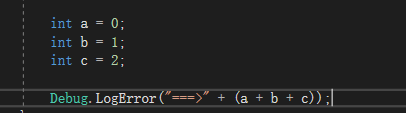如上图，这里有三个局部变量分配了存储空间，所以f(n) = 1 + 1 + 1 = 3，根据上面的法则该函数不受n的影响且为常数项，所以空间复杂度记作O(1)。这种与问题的大小无关（n的多少），执行时间恒定的算法，我们称之为具有O(1)的空间复杂度，又叫常数阶。
2.空间算法的线性阶（递归算法）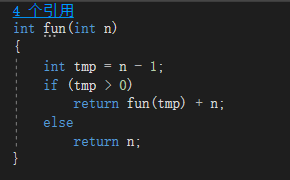如上图，这是一个递归算法（计算从n + (n-1) + (n-2) + … + 2 + 1的和） 每当执行一次该函数就会为tmp分配一个临时存储空间，所以f(n) = 1*(n-1+1) = n，函数是受n影响的所以空间复杂度记为O(n)。
3、二分查找分析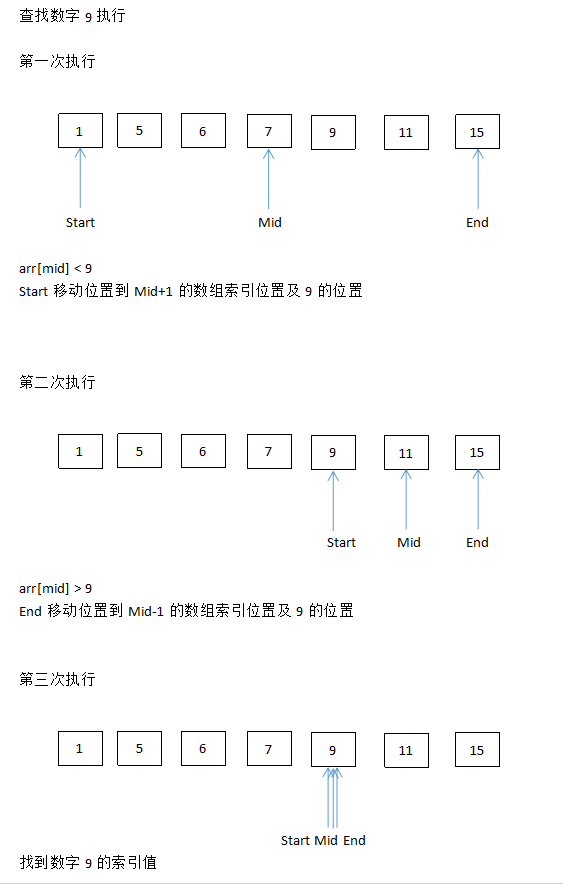方法一（迭代法）：
/// <summary>
/// 二分查找
/// </summary>
/// <param name="arr">查找数组</param>
/// <param name="len">数组长度</param>
/// <param name="num">查找项</param>
/// <returns></returns>
int BinarySearch(int[] arr,int len,int num)
{
int left = 0;
int right = len - 1;
int mid;
while (left <= right)
{
mid = (left + right) / 2;
if (arr[mid] > num)
right = mid - 1;
else if (arr[mid] < num)
left = mid + 1;
else
return mid;
}
return -1;
}

时间复杂度： left、right、mid运算次数 f(n1) = 1 + 1 + 1 = 3 我们将While循环中的运算作为一个整体看待，每次都是折半运算次数 f(n2) = log2^n 总运行次数 f(all) = f(n1)+f(n2) = 3 + log2 ^ n 时间复杂度记为:O(log2^n)

空间复杂度： 算法中left、right、mid只创建的次数 s(n) = 1 + 1 + 1 = 3 空间复杂度记为:O(1)

方法二（递归法）：
 /// <summary>
/// 二分查找(递归法)
/// </summary>
/// <param name="arr"></param>
/// <param name="left"></param>
/// <param name="right"></param>
/// <param name="num"></param>
/// <returns></returns>
int BinarySearchRecursion(int[] arr,int left,int right,int num)
{
int mid = (left + right) / 2;
if (left <= right)
{
if (arr[mid] > num) {
right = mid - 1;
return BinarySearchRecursion(arr,left,right,num);
}
else if (arr[mid] < num)
{
left = mid + 1;
return BinarySearchRecursion(arr,left,right,num);
}
else
return mid;
}
else
{
return -1;
}
}

时间复杂度： 运行次数 f(n) = log2 ^ n 时间复杂度记为:O(log2^n)

空间复杂度： 因为整个算法中mid只创建的次数 s(n) = log2 ^ n 空间复杂度记为:O(log2 ^ n)

4、斐波那契数列
斐波那契数列（Fibonacci sequence），又称黄金分割数列、因数学家列昂纳多·斐波那契（Leonardoda Fibonacci）以兔子繁殖为例子而引入，故又称为“兔子数列”，指的是这样一个数列：1、1、2、3、5、8、13、21、34、…… 这个数列从第3项开始，每一项都等于前两项之和。
如果设F(n）为该数列的第n项（n∈N*），那么这句话可以写成如下形式：:F(n)=F(n-1)+F(n-2)
显然这是一个线性的递推数列。 通项公式 ：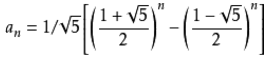上面就是斐波那契数列的递推公式，这样一个完全是自然数的数列，通项公式却是用无理数来表达的。而且当n趋向于无穷大时，前一项与后一项的比值越来越逼近黄金分割0.618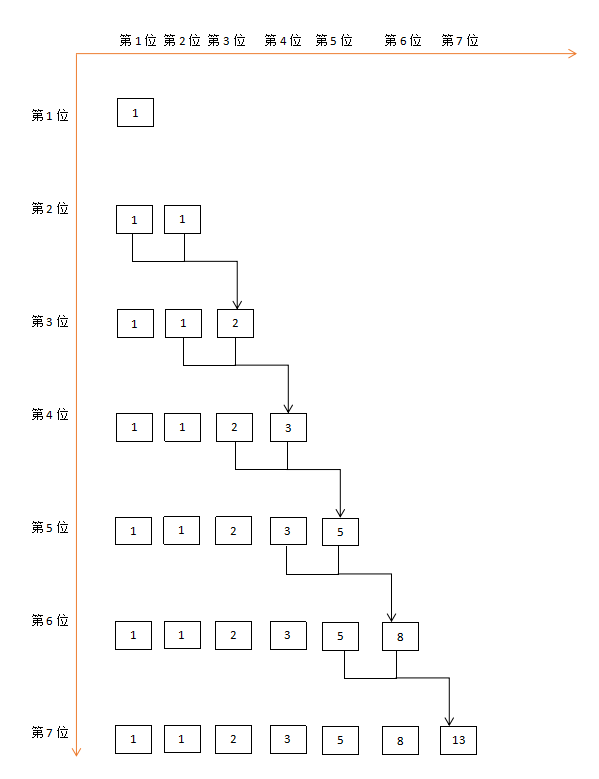递推是公式是求解斐波那契数列的一个方法，我们当然也可以用计算机编写程序来求解。
方法一（迭代法）：
/// <summary>
/// 斐波那契（迭代法）
/// </summary>
/// <param name="n"></param>
/// <returns></returns>
int Fibonacci(int n)
{
if (n <= 0)
return -1;
if (n == 1 || n == 2)
return 1;
else
{
int num = 0;
int a = 1;
int b = 1;
while (n - 2 > 0)
{
num = a + b;
a = b;
b = num;
n--;
}
return num;
}

}

时间复杂度： while以外的算法语句都忽略不计(不随n的变化而变化) while算法语句所有语句 f(n) = 4 *（n - 2） = 4n - 8 时间复杂度记为:O(n)

空间复杂度： 算法中num、a、b只创建1次 s(n) = 1 + 1 + 1 = 3 空间复杂度记为:O(1)

方法二（递归法）：
/// <summary>
/// 斐波那契（递归法）
/// </summary>
/// <param name="n"></param>
/// <returns></returns>
int FibonacciRecursion(int n)
{
if (n <= 0)
return -1;
if (n == 1 || n == 2)
return 1;
return FibonacciRecursion(n - 1) + FibonacciRecursion(n - 2);
}

时间复杂度： 递归调用的形参有两个n - 1 和 n - 2 时间复杂度记为:O(2^n)

空间复杂度： 递归的空间复杂度 =（n + 1）* 调用的深度 空间复杂度记为:O(n)（这里可以简单的根据二叉树的层来进行计算）

展开全文算法 时间复杂度 斐波那契数列
• 常见的时间复杂度计算举例三、空间复杂度 一、算法效率 ???? 如何衡量一个算法的好坏 ????递归代码 ———— 斐波那契数列的代码量十分简洁，所以这个算法是很优的？但其实使用递归是非常戳的，你会发现递归去计算...


文章目录
一、算法效率💦 如何衡量一个算法的好坏💦 算法的复杂度
二、时间复杂度💦 什么是时间复杂度💦 大O渐进表示法 (估算)💦 常见的时间复杂度计算举例💦 常见的复杂度对比💦 根据对时间复杂度的要求编写代码
三、空间复杂度💦什么是空间复杂度💦 常见的空间复杂度计算举例

一、算法效率
💦 如何衡量一个算法的好坏
🎗递归代码 ———— 斐波那契数列的代码量十分简洁，所以这个算法是很优的？但其实使用递归是非常戳的，你会发现递归去计算第40位斐波那契数时都要跑半天，究其原因是内部产生大量重复的计算。那该如何去衡量算法的优劣呢？
#define _CRT_SECURE_NO_WARNINGS
#include<stdio.h>
int Fib(int n)
{
if(n > 2)
return Fib(n - 1) + Fib(n - 2);
else
return 1;
}
int main()
{
int n = 0;
scanf("%d", &n);
int ret = Fib(n);
printf("第%d个斐波那契数是%d\n", n, ret);
return 0;
}

💦 算法的复杂度
算法在编写成可执行程序后，运行时需要耗费时间资源和空间(内存)资源。因此衡量一个算法的好坏，一般是从时间和空间两个维度来衡量的，即时间复杂度和空间复杂度。
时间复杂度主要衡量一个算法的运行快慢，而空间复杂度主要衡量一个算法运行所需要的额外空间。在计算机发展的早期，计算机的存储容量很小。
所以对空间复杂度比较在乎。但是经过计算机行业的迅速发展，计算机的存储容量已经达到了很高的程度。所以我们如今已经不需要再特别关注算法的空间复杂度。

二、时间复杂度
💦 什么是时间复杂度
在计算机科学中，算法的时间复杂度是一个函数，它描述了该算法的运行时间。一个算法执行所耗费的时间，从理论上说，是不能算出来的，只有你把你的程序放在机器上跑起来，才能知道。但是我们需要每个算法都上机测试吗?是可以上机测试，但是这很麻烦，所以才有了时间复杂度这个分析方式。
一个算法所花费的时间与其中语句的执行次数成正比，所以算法中的基本操作的执行次数，为算法的时间复杂度。
即找到某条基本语句与问题规模N之间的数学表达式，就是算出了该算法的时间复杂度。

🎗 计算fun1中++count语句总共执行了多少次
void Func1(int N)
{
int count = 0;
for (int i = 0; i < N ; ++ i)
{
for (int j = 0; j < N ; ++ j)
{
++count;
}
}
for (int k = 0; k < 2 * N ; ++ k)
{
++count;
}
int M = 10;
while (M--)
{
++count;
}
printf("%d\n", count);
}

📝 分析： 从上述代码中可以看出Func1的时间复杂度函数为F(N) = N * N + 2 * N + 10
▶ N = 10    F(N) = 130
▶ N = 100     F(N) = 10210
▶ N = 1000   F(N) = 1002010
💨 从上述就可以看出N越大，对结果的影响就越小。实际中我们计算时间复杂度时，我们其实并不一定要计算精确的执行次数，而只需要大概执行次数，那么这里我们使用大O的渐进表示法 (估算)。
💦 大O渐进表示法 (估算)

大O符号 (Big O notation)：用于描述函数渐近行为的数学符号

🎗推导大O阶的方法：
1️⃣ 用常数１取代运行时间中的所有加法常数
2️⃣ 在修改后的运行次数函数中，只保留最高项
3️⃣ 如果最高阶项存在且系数不是１，则去除与这个项相乘的系数，得到的结果就是大O阶
💨 对于上面的Func1函数，使用大O的渐近表示法后，时间复杂度为O(N^2)
▶ N = 10    F(N) = 100
▶ N = 100     F(N) = 10000
▶ N = 1000   F(N) = 1000000
🎗另外有些算法的时间复杂度存在最好，平均和最坏情况: 例如：在一个长度为N的数组中查找一个数据X，最好的情况1次就找到；平均的情况N/2就找到；最坏的情况N次才找到
1️⃣ 最坏情况:任意输入规模的最大运行次数(上界)
2️⃣ 平均情况:任意输入规模的期望运行次数
3️⃣ 最好情况:任意输入规模的最小运行次数(下界)
💨 在实际中一般情况关注的是算法的最坏运行情况，所以数组中搜索数据时间复杂度为O(N)
💦 常见的时间复杂度计算举例
🎗实例1:
void Func2(int N)
{
int count = 0;
for (int k = 0; k < 2 * N ; ++ k)
{
++count;
}
int M = 10;
while (M--)
{
++count;
}
printf("%d\n", count);
}

📝 分析： Func2的时间复杂度函数为F(N) = (2N + 10) 使用大O渐近表示法：保留影响最大的一项、去掉系数则为O(N)
🎗实例2:
void Func3(int N, int M)
{
int count = 0;
for (int k = 0; k < M; ++ k)
{
++count;
}
for (int k = 0; k < N ; ++ k)
{
++count;
}
printf("%d\n", count);
}

📝 分析： Func3的时间复杂度函数为F(N) = (M + N) 使用大O渐近表示法：不一定只有一个未知数，所以这里可以写O(M + N) 也可以写成如下： ▶ O(ｍax(M, N))：取M和N的较大值
▶ O(M)：如果能说明M远大于N
▶ O(N)：如果能说明N远大于M
▶ O(N)/O(M)：如果能说明M和N差不多大
🎗实例3:
void Func4(int N)
{
int count = 0;
for (int k = 0; k < 100; ++k)
{
++count;
}
printf("%d\n", count);
}

📝 分析： Func4的时间复杂度函数为F(N) = (100)  使用大O渐近表示法：使用１代表常数，所以O(1)

🎗实例4:
void BubbleSort(int* a, int n)
{
assert(a);
for (size_t end = n; end > 0; --end)
{
int exchange = 0;
for (size_t i = 1; i < end; ++i)
{
if (a[i-1] > a[i])
{
Swap(&a[i-1], &a[i]);
exchange = 1;
}
}
if (exchange == 0)
break;
}
}

📝 分析： 这是冒泡排序的一个优化版本，在一趟排序的过程中如果没有交换数据的话，它就会跳出循环，所以它是有最好、平均、最坏的情况的 BubbleSort的时间复杂度函数为F(N) = (n + (n - 1) + (n - 2) … + 2 + 1) 所以你会发现这是一个等差数列，利用公式整合得：F(N) = (n + 1)* n / 2 -> F(N) = n^2 / 2 + n / 2 使用大O渐近表示法： (最坏情况)：O(N^2) -> 这是我们要考虑的情况，显然如果是最坏的情况，那我们就优化了个寂寞           (平均情况)：O(N^2) -> (n^2 / 2 + n / 2)/2           (最好情况)：O(N)
🎗实例4:
int BinarySearch(int* a, int n, int x)
{
assert(a);
int begin = 0;
int end = n-1;
while (begin < end)
{
int mid = begin + ((end-begin)>>1);
if (a[mid] < x)
begin = mid+1;
else if (a[mid] > x)
end = mid;
else
return mid;
}
return -1;
}

📝 分析： BinarySearch依然存在最好、平均、最坏的情况： BinarySearch的时间复杂度函数为F(N) = N / 2 / 2 / 2 … /2 = 1 使用大O渐近表示法：O(log₂N)或O(logN) -> 因为底数不好打出来，有时候一般也这样写 1、N / 2 2、N / 2 / 2 -> N / 4：N / 2^2 3、N / 2 / 2 / 2 -> N / 8：N / 2^3 x、N / 2^x = 1 -> N = 2^x -> log₂N = x
🎗实例5:
long long Fac(size_t N)
{
if(1 == N)
return 1;
return Fac(N-1)*N;
}

📝 分析： Fac的时间复杂度为F(N) = (N) 使用大O渐近表示法：O(N)
🎗实例6:
long long Fib(size_t N)
{
if(N < 3)
return 1;
return Fib(N-1) + Fib(N-2);
}

📝 分析：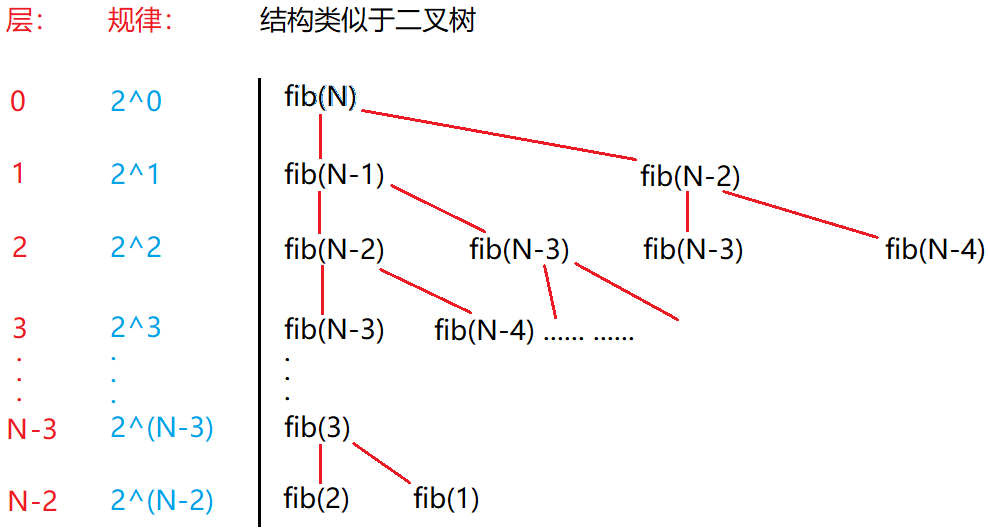2^0 + 2^1 + 2^2 + 2^3 … +2^(N-3) + 2^(N-2)
使用大O渐近表示法：O(2^N)
💦 常见的复杂度对比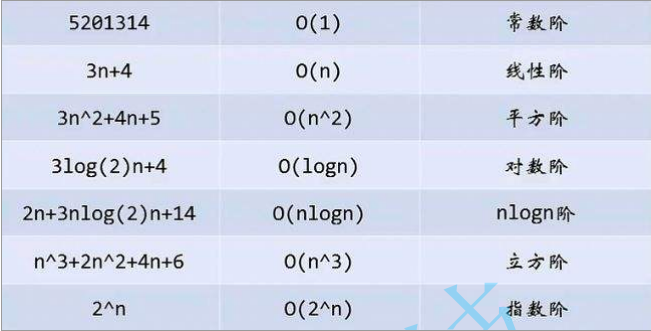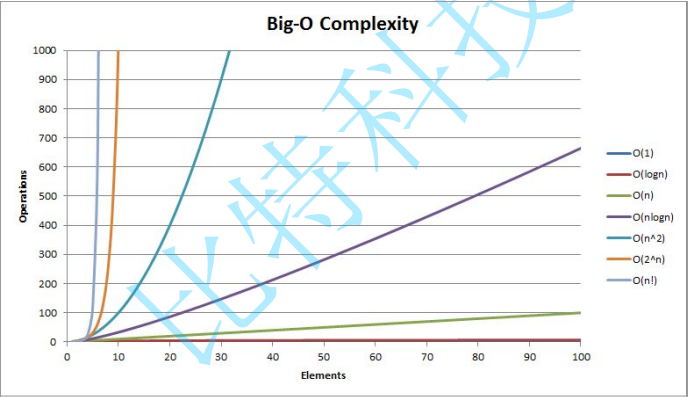💦 根据对时间复杂度的要求编写代码
🎗实例1:消失的数字 📝 题述：数组arr包含从０到ｎ的所有整数，但其中缺了一个，编写代码找出那个缺失的整数，时间复杂度限制为O(N) 💨 输入描述：输入０到ｎ的整数，并少输一个数 💨 输出描述：输出那个少输的数 🔑 核心思想： 方法一：先排序，再依次判断第１个数和之后的数相加是否等于第３个数，若不等，则它们的和就是缺失的数————冒泡排序时间复杂度O(N²)，快速排序时间复杂度O(N*log₂N) 方法二：求和，如果有n个数，则０＋１＋２…＋ｎ，最后整体再减去数组中的值的累加就是缺失的数————时间复杂度O(N) 方法三：异或，使用０跟０—ｎ之间的数异或，再跟数值中的值异或，异或的结果就是缺失的数 🎗 方法2
#define _CRT_SECURE_NO_WARNINGS
#include<stdio.h>
int FindNum1(int arr[], int n)
{
int i = 0;
//把n+1个数加起来，放在sum里
int sum = 0;
for (i = 0; i < n + 1; i++)
{
sum += i;
}
//再减去数组里的数，结果就是缺失的数
for (i = 0; i < n; i++)
{
sum -= arr[i];
}
return sum;
}
int main()
{
int arr = { 0 };
//规定输入有n个数
int n = 3;
int i = 0;
for (i = 0; i < n; i++)
{
scanf("%d", &arr[i]);
}
int ret = FindNum1(arr, n);
printf("%d\n", ret);
return 0;
}

🎗 方法3
#define _CRT_SECURE_NO_WARNINGS
#include<stdio.h>
int FindNum2(int arr[], int n)
{
int i = 0;
//把n+1个数异或后，放在sum里
int sum = 0;
for (i = 0; i < n + 1; i++)
{
sum ^= i;
}
//再和数组里的异或，剩下的就是缺失的数
for (i = 0; i < n; i++)
{
sum ^= arr[i];
}
return sum;
}
int main()
{
int arr = { 0 };
//n个数
int n = 3;
int i = 0;
for (i = 0; i < n; i++)
{
scanf("%d", &arr[i]);
}
int ret = FindNum2(arr, n);
printf("%d\n", ret);
return 0;
}

🎗实例2:旋转字符串 📝 题述：给定一个数组，将数组中的元素向右移动 k 个位置，其中 k 是非负数。要求时间复杂度O(N) 💨 输入描述：输入n个字符，输入要右移的k个位置 💨 输出描述：输出右移后的数组 🔑 核心思想： 方法一：这是１个字符的旋转，拷贝一份最右值，数组中的值都向右挪劫１次，再把拷贝的内容放在开头；在外面套一层循环就可以旋转k个字符了————时间复杂度O(N²)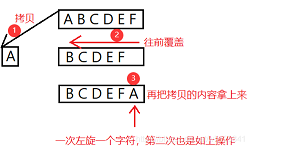方法二：空间换时间————时间复杂度O(N)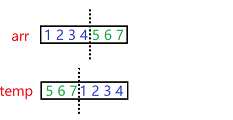方法三：三步翻转法————时间复杂度O(N)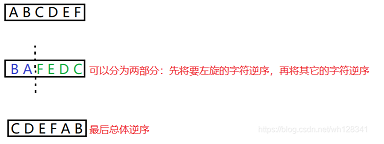🎗 方法2
#define _CRT_SECURE_NO_WARNINGS
#include<stdio.h>
#include<string.h>
#include<assert.h>
char* Rotate1(const char* arr, int len, int k, char* temp)
{
assert(arr && temp);
//拷贝一份新空间的首地址用于返回
char* tem = temp;
//我们当前写的这个代码是不适用于旋转的字符k大于目标数组的arr，所以如果k大于arr时，我们需要看看k有几个arr,并把它排除掉
k %= len;
int i = 0;
//先拷贝后半部分的字符
for (i = len - k; i < len; i++)
{
*temp = *(arr + i);
temp++;
}
//再拷贝前半部分的字符
for (i = 0; i < len - k; i++)
{
*temp = *(arr + i);
temp++;
}
return tem;
}
int main()
{
//temp为新的空间
char temp = { 0 };
//arr存储要旋转的字符串
char arr = { 0 };
gets(arr);
//旋转k个字符
int k = 0;
scanf("%d", &k);
char* ret = Rotate1(arr, strlen(arr), k, temp);
printf("%s\n", ret);
return 0;
}

🎗 方法３
#define _CRT_SECURE_NO_WARNINGS
#include<stdio.h>
#include<assert.h>
#include<string.h>
void reverse(char* left, char* right)
{
assert(left && right);
while (left < right)
{
char temp = *left;
*left = *right;
*right = temp;
left++;
right--;
}
}
void string_right_rotation(char* str, int k)
{
assert(str);
int len = strlen(str);
//我们当前写的这个代码是不适用于旋转的字符k大于目标数组的arr，所以如果k大于arr时，我们需要看看k有几个arr,并把它排除掉
k %= len;
reverse(str, str + (len - k - 1));//第一部分
reverse(str + (len - k), str + len - 1);//第二部分
reverse(str, str + len - 1);//整体
}
int main()
{
//arr存储要旋转的字符串
char arr = { 0 };
gets(arr);
//旋转k个字符
int k = 0;
scanf("%d", &k);
string_right_rotation(arr, k);
printf("%s\n", arr);
return 0;
}

三、空间复杂度
💦什么是空间复杂度
空间复杂度也是一个数学表达式，是对一个算法在运行过程中临时占用存储空间大小的量度。
空间复杂度不是程序占用了多少bytes的空间，因为这个也没太大意义，所以空间复杂度算的是变量的个数。空间复杂度计算规则基本跟时间复杂度类似，也使用大o渐进表示法。
注意:函数运行时所需要的栈空间(存储参数、局部变量、一些寄存器信息等)在编译期间已经确定好了，因此空间复杂度主要通过函数在运行时候显式申请的额外空间来确定。

💦 常见的空间复杂度计算举例
相对简单，过一下即可： 🎗实例1:
void BubbleSort(int* a, int n)
{
assert(a);
for (size_t end = n; end > 0; --end)
{
int exchange = 0;
for (size_t i = 1; i < end; ++i)
{
if (a[i-1] > a[i])
{
Swap(&a[i-1], &a[i]);
exchange = 1;
}
}
if (exchange == 0)
break;
}
}

📝 分析： 相比时间复杂度来说：时间是累计的，但空间不是累计的(可以重复利用) BubbleSort的空间复杂度为F(N) = (３) 使用大O渐近表示法：O(１)
🎗实例2:
long long* Fibonacci(size_t n)
{
if(n==0)
return NULL;

long long * fibArray = (long long *)malloc((n+1) * sizeof(long long));
fibArray = 0;
fibArray = 1;
for (int i = 2; i <= n ; ++i)
{
fibArray[i] = fibArray[i - 1] + fibArray[i - 2];
}
return fibArray;
}

📝 分析： 使用大O渐近表示法：O(N)
🎗实例3:
long long Fac(size_t N)
{
if(N == 1)
return 1;
return Fac(N-1)*N;
}

📝 分析： 使用大O渐近表示法：O(N)
🎗实例1:
long long Fib(size_t N)
{
if(N < 3)
return 1;
return Fib(N-1) + Fib(N-2);
}

📝 分析： 使用大O渐近表示法：O(N)展开全文• 文章目录算法的时间复杂度和空间复杂度复杂度的分析一. 时间维度事后统计法事前分析估算的方法时间复杂度(1)时间频度(2)时间复杂度大O符号表示法常见的时间复杂度量级常数阶O(1)线性阶O(n)对数阶O(logN)线性对数阶O...c++ 算法
• 递归的时间复杂度三、空间复杂度1.空间复杂度概念2.空间复杂度的计算(1) 冒泡排序(2) 斐波那契数列(3)递归总结 一、算法效率 算法效率分析分为两种：第一种是时间效率，第二种是空间效率。时间效率被称为时间复杂度...java 算法 数据结构
• 时间复杂度和空间复杂度前言算法效率时间复杂度1.时间复杂度的概念2.大O的渐进（估算）表示法推导大O阶方法(重点！！！)：另外有些算法的时间复杂度存在最好、平均、和最坏情况（重点！！！）：3.常见时间复杂度计算...数据结构 算法 c语言
• ## 简析时间复杂度和空间复杂度

万次阅读 多人点赞 2019-06-25 16:24:17
时间复杂度和空间复杂度是用来评价算法效率高低的2个标准，身为开发者肯定会经常会听到这2个概念，但它们分别是什么意思呢? 其实这两个概念从字面意思上也能看出一二： 时间复杂度：就是说执行算法需要消耗的时间...时间复杂度
• 空间复杂度(Space Complexity)记作S(n) 依然使用大O来表示利用程序的空间复杂度，可以对程序运行时所需要多少内存有个预先估计。我这里来回答两个常见的相关问题空间复杂度是考虑程序(可执行文件)的大小么？...
• 时间复杂度和空间复杂度详解 目录1.算法效率2.时间复杂度3.空间复杂度 1.算法效率 算法效率分析分为两种：第一种是时间效率，第二种是空间效率。时间效率被称为时间复杂度，而空间效率被称作空间复杂度。 时间复杂度...数据结构 算法
• 复杂度分析主要就是时间复杂度和空间复杂度，接下来的文章也主要围绕这两方面来讲。废话不多说，前排马扎瓜子准备好，蛋蛋小课堂正式接客图片 复杂度分析 刚刚我说过，在本蛋看来，复杂度分析是数据结构和算法中最...数据结构与算法 时间复杂度
• 归并排序的时间复杂度任何情况下都是 O(nlogn)，看起来非常优秀。...那我现在问你，归并排序的空间复杂度到底是多少呢？是 O(n)，还是 O(nlogn)，应该如何分析呢？ 如果我们继续按照分析递归时间复归并排序 数据结构
• 干货文章，作者花了四个小时为大家整理了这篇干货文章，希望大家认真阅读，本文可能难度较大，建议配合网络上搜索的其他资源来阅读，作者大大：刘权欣同学
• 一般算法的空间复杂度相信大家已经都掌握了 那么大家想一想递归算法的空间复杂度应该怎么分析呢。 我这里用一个到简单的题目来举例 题目：求第n的斐波那契数 相信使用递归算法来求斐波那契数，大家应该再熟悉不过了 ...数据结构和算法 递归算法
• 1、算法时间复杂度 1.1算法时间复杂度的定义：  在进行算法分析时，语句总的执行次数T(n)是关于问题规模n的函数，进而分析T(n)随n的变化情况并确定T(n)的数量级。算法的时间复杂度，也就是算法的时间量度，记作...数据结构 时间复杂度 算法
• ## 快速理解时间复杂度与空间复杂度

千次阅读 多人点赞 2018-05-11 17:48:05
1.首先，时间复杂度与空间复杂度是对立的，时间复杂度越低，空间复杂度就越高。我们在程序中通常采用以空间换时间的方式来提高项目运行效率。 先给大家说一个最常见的简单的，时间复杂度是以n为变量，程序的运行次数...
• 需要引入一个衡量的标准(metric)—时间复杂度和空间复杂度。 学习数据结构和算法的基石，就是要学会复杂度分析。知道怎么去分析复杂度，才能作出正确的判断，在特定的场景下选用合适的正确的算法。而不是盲目的死记...算法 数据结构 java python 机器学习
• 其中，上面提到的效率可以用算法的时间复杂度来描述，而所占用的存储空间可以用算法的空间复杂度来描述。 时间复杂度：用于评估执行程序所消耗的时间，可以估算出程序对处理器的使用程度。 空间复杂度：用于评估执行...java
• 时间复杂度、空间复杂度，如果要严格意义的数学证明的话，公式比较繁复，这些公式很让人头疼，我们简单地通过读程序来判断时间复杂度。 时间复杂度的表达： Big O notation 常见的7种时间复杂度： O(1):Constant...算法 数据结构
• 目录 概念 复杂度分析 时间复杂度分析 空间复杂度分析 ...算法复杂度：是指算法在编写成可执行程序后，运行时所需要的资源，包括时间资源（运行算法耗费的时间）和内存资源（程序运行占用的...空间复杂度：一个算...时间复杂度 算法复杂度 算法效率分析 大O表示法
• 6.怎么在时间复杂度O(1)，空间复杂度O(1)下计算斐波那契数 1.斐波那契数简介 斐波那契数列（Fibonacci sequence），又称黄金分割数列、因数学家莱昂纳多·斐波那契（Leonardoda Fibonacci）以兔子繁殖为例子而...
• 算法复杂度分为时间复杂度和空间复杂度。其作用： 时间复杂度是度量算法执行的时间长短；而空间复杂度是度量算法所需存储空间的大小。1、时间复杂度1.1 时间频度一个算法中的语句执行次数称为语句频度或时间频度。记......